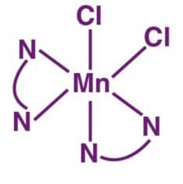# Total number of  N – Mn$-$ Cl (adjacent) bond angles in cis form  of  $\left[\mathrm{Mn}{\left(\mathrm{en}\right)}_{2}{\mathrm{Cl}}_{2}\right]$ complex is.......... (en= ${\mathrm{NH}}_{2}{\mathrm{CH}}_{2}{\mathrm{CH}}_{2}{\mathrm{NH}}_{2}\right)$

FREE Lve Classes, PDFs, Solved Questions, PYQ's, Mock Tests, Practice Tests, and Test Series!

+91

Verify OTP Code (required)

I agree to the terms and conditions and privacy policy.

### Solution:

This is the cis form of $\left[\mathrm{Mn}{\left(\mathrm{en}\right)}_{2}{\mathrm{Cl}}_{2}\right]$Therefore No of N – Mn – Cl bonds = 6

## Related contentJoin Infinity Learn Regular Class Program!

Sign up & Get instant access to FREE PDF's, solved questions, Previous Year Papers, Quizzes and Puzzles!

+91

Verify OTP Code (required)

I agree to the terms and conditions and privacy policy.